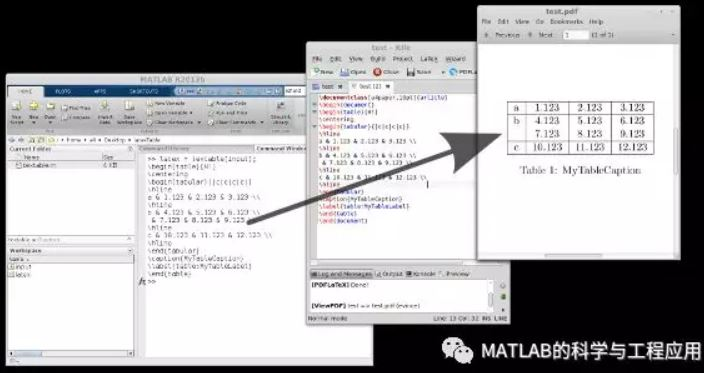• matlab 表格建立的过程，还有相应的一些属性描述
• 一个 MATLAB 函数，它从 Excel 文件中读取内容并将其转换为 LaTeX 表格代码，或者将 MATLAB 表格或元胞数组转换为 LaTeX 表格代码。 LaTeX 表代码指的是由 & 分隔的列条目和以 \\ 结尾的行
• 本代码将矩阵、单元格和MATLAB表格转换为LaTex表格代码。 Converts matrices, cells or MATLAB tables to LaTeX table code. 本代码具有易用和灵活的特点： Easy to use and very flexible: MATLAB矩阵、单元格或者...本代码将矩阵、单元格和MATLAB表格转换为LaTex表格代码。
Converts matrices, cells or MATLAB tables to LaTeX table code.
本代码具有易用和灵活的特点：
Easy to use and very flexible:
MATLAB矩阵、单元格或者表格作为输入

takes MATLAB matrices, cells or tables as input

提供包含数据作为输出的LaTeX表代码

gives code for a LaTeX table containing your data as output

处理数字和文本/字符串数据

handles numeric and text/string data

很容易将其包含在自动化设计代码中

easy including in your code for automation

易于直接保存为LaTex文档（请参见runExamples.m中的示例2）

easy direct saving as a LaTex document (see example 2 in runExamples.m)

其它特点包括：
Optional features are:
表格定位

table positioning

列/行标记

column/row labels

列/行格式设置（如设置数字的位数等）

column/row-wise formatting (like setting the number of digits etc.)

表格旋转（通过90度旋转对行列数据进行反转）

pivoting the table (90 degree rotation making columns rows and vice versa)

对NaN数据设置缺省的符号

setting the default symbol for NaN values

LaTeX列对齐

LaTeX column alignment

LaTeX表格边框、标题、标签

LaTeX table borders, caption, label

输出完整的LaTeX文档，而不是普通表格

output a complete LaTeX document instead of a plain table

示例：
input.data=[1,3;4,2];             % set up data
latexTable(input)                 % obtain LaTeX code
更多的细节和示例可以在latexTable.m和runExamples.m中找到。
For further details and examples can be found in latexTable.m and runExamples.m
源码下载地址：
http://page2.dfpan.com/fs/clac2jc2e211f259160/
更多精彩文章请关注微信号：展开全文• 输入纸质表格，输出excel文件 主程序输入I=imread(‘文件名’)的图片，I1为矩阵，再使用xlwrite(‘文件名’,I1)，得到excel文件。 需要预先训练好一个CNN网络(看matlab深度学习帮助文档），保存为et.mat。 效果 %...
输入纸质表格，输出excel文件
主程序输入I=imread(‘文件名’)的图片，I1为矩阵，再使用xlwrite(‘文件名’,I1)，得到excel文件。
需要预先训练好一个CNN网络(看matlab深度学习帮助文档），保存为et.mat。
效果%主程序 excel_id.m
function I1=excel_id(I)
global fi;
fi=0;
load 'et.mat';%加载CNN

I=cutall(I);%变黑白，去除空白
for i=1:10
if length(I)<=400
break;
end
I=imresize(I,0.9);%缩小图片
end
PA=cutline3(I);%分割成一个个单元格
[y,x]=size(PA);

for i=1:x
for j=1:y
PA2 = num_cut2(suo2(PA{j,i}));%提取一个个字符
sum=calcu(PA2,et);%识别字符，连起来
output{j,i}=sum;%保存结果
end
end
I1=output;
end

%去除空白部分
function T = cutall(I)
I=im2bw(I,0.43);%变黑白
[y, x]=size(I);
y2=floor(y/2);x2=floor(x/2);%定位四个点，提取表格主要部分
for i=1:x
if I(y2,i)==0
cut11=i;
break
end
end
for i=1:x
if I(y2,x+1-i)==0
cut12=x+1-i;
break
end
end
for j=1:y
if I(j,x2)==0
cut21=j;
break
end
end
for j=1:y
if I(y+1-j,x2)==0
cut22=y+1-j;
break
end
end
T=I(cut21:cut22,cut11:cut12);
end

%分割成一个个单元格
function PA = cutline4(I)
%I=imread('49-3-BW01.jpg');
[y, x]=size(I);
p=sum(I);
I1=I';
q=sum(I1);%计算水平和垂直投影
signnew=1;sign=1;
for i=floor(0.05*x):floor(0.95*x)
if((i-signnew)>floor(0.05*x))
if p(i) <= 0.4*y
sign=[sign i];%记录符合的点
signnew=i;
end
end
end
sign=[sign x];
[H11,W11]=size(sign);
signnew2=1;sign2=1;
for j=floor(0.05*y):floor(0.95*y)
if((j-signnew2)>floor(0.05*y))
if q(j) <= 0.4*x
sign2=[sign2 j];%记录符合的点
signnew2=j;
end
end
end
sign2=[sign2 y];
[H22,W22]=size(sign2);
PA=cell(W22-1,W11-1);
se=strel('square',2);
I=imerode(I,se);
I=qubiankuang(I);%去边框
for i=1:(W11-1)
for j=1:(W22-1)
PA{j,i}=I(sign2(1,j):sign2(1,j+1),sign(1,i):sign(1,i+1));%分割
end
end
end

%去除边框
function I1=qubiankuang(I)
I=im2bw(I);
I1=imcomplement(I);
[ L, num]=bwlabel(I1,8);%连通域标记
x=find(L==1);%找出边框所在位置
for i=x
I1(i)=0;%去除边框
end
I1=imcomplement(I1);
end

%剪去边缘
function s=suo2(Img)
[y, x]=size(Img);
s4=floor(0.95*y);
s3=ceil(0.1*y);
s2=floor(0.95*x);
s1=ceil(0.01*x);%减去边缘

Img=Img(s3:s4,s1:s2);
s=Img;
end

%分离出一个个字符
function PA2 = num_cut2(Img)
global fi;
Img=im2uint8(Img);
[y, x]=size(Img);
[~,~,c]=size(Img);
if  c==1
f2= ~im2bw(Img);
else
Img=rgb2gray(Img);
f2= ~im2bw(Img);
end
f=f2;
f=bwareaopen(f,50);
str=strel('square',2);
f=imdilate(f,str);
fi=fi+1;figure(fi);
imshow(f);
[L,num] = bwlabel(f,8);%标注二进制图像中已连接的部分
Feastats = regionprops(L,'basic');%计算图像区域的特征尺寸
Area=[Feastats.Area];%区域面积
b=[Feastats.BoundingBox];%[x y width height]字符的大小
sub=cell(1,num);%存储分割后的图像

fi=fi+1;figure(fi);
%分割过程
for i=1:num
a=floor(b((i-1)*4+2));%b中字符的特性是按4个一组放的，前两个为左上顶点坐标，后两个为长宽。
if a==0
a=1;
end
e=b((i-1)*4+4);
d=floor(b((i-1)*4+1));

if d==0
d=1;
end
g=b((i-1)*4+3);
if( g<=0.8*x && e<=0.95*y)
sub{1,i}=f2(a:a+e,d:d+g);%取出字符块
subplot(1,num,i);imshow(sub{1,i});
end
end
hold on
for k=1:num
[r,c]=find(L==k);
rbar=mean(r);
cbar=mean(c);    plot(cbar,rbar,'Marker','o','MarkerEdgeColor','k','MarkerFaceColor','k','MarkerSize',10);    plot(cbar,rbar,'Marker','*','MarkerEdgecolor','w');
end
PA2=sub;
end

%对每个单元格识别出结果
function num = calcu(PA2,net)
[y,x]=size(PA2);
y1m=1;
for i=1:x
[y1,x1]=size(PA2{1,i});
if y1>y1m
y1m=y1;%计算最大字符高度
end
end
n=0;si=[];
for i=1:x
[y1,x1]=size(PA2{1,i});
if(y1 > floor(0.3*y1m))%防止过小的物体被当做字符
n=n+1;
si=[si i];
end
end
PA3=cell(1,n);
for i=1:n
PA3{1,i}=PA2{1,si(i)};
end
PA2=PA3;
[y,x]=size(PA2);
sum=[];
for i=1:x
imin=Tresize(PA2{1,i});%缩放图片使其符合CNN输入尺寸
pred=classify(net,imin);%识别
N=char(pred);
sum=[sum N];%保存结果
end
num=sum;
end

%缩放图片使其符合CNN输入
function T = Tresize(T1)
T=imcomplement(T1);
[y, x]=size(T);
AD=ones(floor(0.1*y),x);
T=[AD; T; AD];
[y, x]=size(T);
AD=ones(y,floor((y-x)/3));
T=[AD T AD];%增添空白区域使其符合输入
T=imresize(T,[16 16]);%缩放
T=im2uint8(T);
end



展开全文图像识别 深度学习
• 制作一个可展示的表格有几个FEX贡献。 这不是其中的一个。 R2013b 中引入了一个非常有用的数据容器，称为表。 本白皮书展示了一些使用表格的中间方法。
• 函数 table2latex(T, filename) 按照 LaTeX 格式将 MATLAB 表 (T) 格式化为 .tex 文件（由文件名路径指定）。 请避免在表格内使用单元格或结构。
• 将输入数组或元胞数组打印为格式良好的表格。 将分隔符设置为 " " 以仅格式化输出而不看起来像表格
• ## matlab表格生成

千次阅读 2018-05-17 18:26:53
figure(3); set(3,'Name','Calibration results','NumberTitle','off'); subplot(2,1,1); ssmy=[-2.224834e+02 ;0.000000e+00 ;1.990932e-03; -4.004428e-06; 1.218167e-08]; ssit=[-2.169126e+02 ;... 1....
figure(3);
set(3,'Name','Calibration results','NumberTitle','off');
subplot(2,1,1);
ssmy=[-2.224834e+02 ;0.000000e+00 ;1.990932e-03; -4.004428e-06; 1.218167e-08];
ssit=[-2.169126e+02 ;0.000000e+00; 1.585854e-03 ;-3.183199e-07; 3.767708e-09];
x=0:0.02*370:370;
% plot(0:floor(374.956334),polyval([ssmy(end:-1:1)],[0:floor(374.956334)]),':',0:floor(369.620933),polyval([ssit(end:-1:1)],[0:floor(369.620933)]),'k.'); grid on; axis equal;
mmh=plot(x,polyval([ssmy(end:-1:1)],[0:0.02*double(370):double(370)]),'*k',x,polyval([ssit(end:-1:1)],[0:0.02*double(370):double(370)]),'ok');legend({'Proposed','Urban'},'Location','NorthWest'); grid off; %axis equal;
% plot(0:floor(374.956334),polyval([ssmy(end:-1:1)],[0:floor(374.956334)]),'-.',0:floor(369.620933),polyval([ssit(end:-1:1)],[0:floor(369.620933)]),'--'); grid on; axis equal;
xlabel('Distance ρ from the image center in pixels');
ylabel('f(ρ)');
title('Forward projection function');
%
% subplot(2,1,2);
% plot(0:floor(calib_data.ocam_model.width/2),180/pi*atan2(0:floor(calib_data.ocam_model.width/2),-polyval([ss(end:-1:1)],[0:floor(calib_data.ocam_model.width/2)]))-90); grid on;
% xlabel('Distance ''rho'' from the image center in pixels');
% ylabel('Degrees');
% title('Angle of optical ray as a function of distance from circle center (pixels)');

展开全文• 然后通过函数“convertCellsToTable()”将数据转换为表格。 允许的数据字段是“char”字符串、一维“double”数组和任意大小的一维“cell”数组。 然后可以使用 writetable() 将该表导出到文本文件。 运行...
• 我要赚分啊。matlab跑出的数据结果.matlab工作空间结果。无错误
• 如何利用matlab根据excel表格里面的数据画图方法/步骤1，在excel中输入制图表所需要的数据。2，选择数据(选择不连续的数据可以按住“ctrl”在选择就可以了。还有选择时要连表头一起选择)。3，在“插入”菜单“图表”...
如何利用matlab根据excel表格里面的数据画图方法/步骤1，在excel中输入制图表所需要的数据。2，选择数据(选择不连续的数据可以按住“ctrl”在选择就可以了。还有选择时要连表头一起选择)。3，在“插入”菜单“图表”中选择所需要的图表，例如要制作三维簇形柱形图，就选择柱形图，三维，簇形，选择就可以了。4，如果觉得制作的图表不够完善，可以在“图表工具”下选择修改，例如增加标题，调整图例位置。如何将excel表格中大量数据导入matlab中并作图"在excel中由数据画图，且显示图线的函数"的操作步骤是：1、以Excel 2016为例，打开Excel工作表；2、选择数据区域，在“插入”选项下的“图表”中，选择“散点图”；3、在图表区域，单击鼠标右键，“添加趋势线”；4、根据默认趋势线与散点的重合程度，默认线性趋势线不符合要求，点击“趋势线”，在“设置趋势线格式”中，勾选趋势线选项为“幂”；5、并勾选“显示公式”和“显示R平方值”，得到根据图表数据所生成的函数表达式，这与自由落体运动的公式相符，＝gt^2/2excel表格怎么手动画图方法/步骤1，在excel中输入制图表所需要的数据。2，选择数据(选择不连续的数据可以按住“ctrl”在选择就可以了。还有选择时要连表头一起选择)。3，在“插入”菜单“图表”中选择所需要的图表，例如要制作三维簇形柱形图，就选择柱形图，三维，簇形，选择就可以了。4，如果觉得制作的图表不够完善，可以在“图表工具”下选择修改，例如增加标题，调整图例位置。如何用excel表格中的数据制图方法/步骤1，在excel中输入制图表所需要的数据。2，选择数据(选择不连续的数据可以按住“ctrl”在选择就可以了。还有选择时要连表头一起选择)。3，在“插入”菜单“图表”中选择所需要的图表，例如要制作三维簇形柱形图，就选择柱形图，三维，簇形，选择就可以了。4，如果觉得制作的图表不够完善，可以在“图表工具”下选择修改，例如增加标题，调整图例位置。我想在excel中由数据画图，且显示图线的函数，怎么..."在excel中由数据画图，且显示图线的函数"的操作步骤是：1、以Excel 2016为例，打开Excel工作表；2、选择数据区域，在“插入”选项下的“图表”中，选择“散点图”；3、在图表区域，单击鼠标右键，“添加趋势线”；4、根据默认趋势线与散点的重合程度，默认线性趋势线不符合要求，点击“趋势线”，在“设置趋势线格式”中，勾选趋势线选项为“幂”；5、并勾选“显示公式”和“显示R平方值”，得到根据图表数据所生成的函数表达式，这与自由落体运动的公式相符，＝gt^2/2
展开全文• ## MATLAB写入表格

千次阅读 2019-10-23 16:34:15
MATLAB写入表格遇到了bug？请看这里......
• MATLAB 表格数据的处理（str,char,datetime） 读取表格数据
• ## MATLAB生成excel表格

万次阅读 多人点赞 2018-11-13 18:26:00
利用excel对数据进行复杂处理有一定局限性，于是选择导入MATLAB进行处理。此贴针对MATLAB将数据导出至excel，列举一些简单的例子，帮助大家学习。excel
• 这个一个m文件，利用Matlab，直接生成latex代码，可直接插入latex中，生成表格，方便快捷
• 可以通过matlab读取excel表格并画图，可以索引至第n列，功能易实现，可验证
• MATLAB读取文件：[num]=csvread('timestamps.csv',1,1) MATLAB保存文件：save timestamp.txt -ascii num（变量名）
• 关于matlab guide 表格编辑器，制作一个可以增删的动态表格 https://blog.csdn.net/u011798443/article/details/82821095
• matlab开发-Matlabtogoogle电子表格matlab函数将数据发送到谷歌电子表格
• 如何从Excel到Matlab读取表格并绘制2列中的值由应用设计师
• 如何用Matlab把excel表格中的数据导入Matlab中并绘制出图？   1.将excel中的数据导入Matlab中，点击“导入数据” 2.弹出“Import”窗口，在窗口工具栏左侧导入选项中选择“Matrix（矩阵）”，然后点击工具栏...
• matlab gui界面设计时，常常用到表格，用于输入或导入数据，从而进行计算。本期将讲解matlab gui的表格控件的设置和使用方法。 首先在gui界面中建立一个表格： 双击表格进入属性界面： 在Data中可以编辑...
• ## Matlab读写excel表格

千次阅读 2018-06-06 09:20:56
利用Matlab可以很方便地对excel内的数据进行读入，并且可以将指定数据写入excel中去。（1）读取excel数据。%% num = xlsread(filename, sheet, xlRange) A = xlsread('dataset.xlsx', 'Sheet1', 'A22:E52');...
• 非常适合初学者的MATLAB操作Excel表格的代码，包括删减表格，移动行列等操作Excel表格的代码
• 该电子表格使在 Excel 和 MATLAB 之间来回传递数据变得容易。 它将范围列表传递给 MATLAB，运行电子表格中列出的代码，然后将变量传递回 Excel 范围。 有运行探查器的选项，以及清除变量的各种选项。 附件是演示...
• Spreadsheet Link:trade_mark: 将 Excel:registered: 电子表格软件与 MATLAB:registered: 工作区连接起来，使您能够从 Excel 电子表格访问 MATLAB 环境。 使用 Spreadsheet Link 软件，您可以在 MATLAB 和 Excel ...
• matlab读取表格，并统计成绩，得出最高分，平均分，和中间的成绩...

# matlab表格matlab 订阅